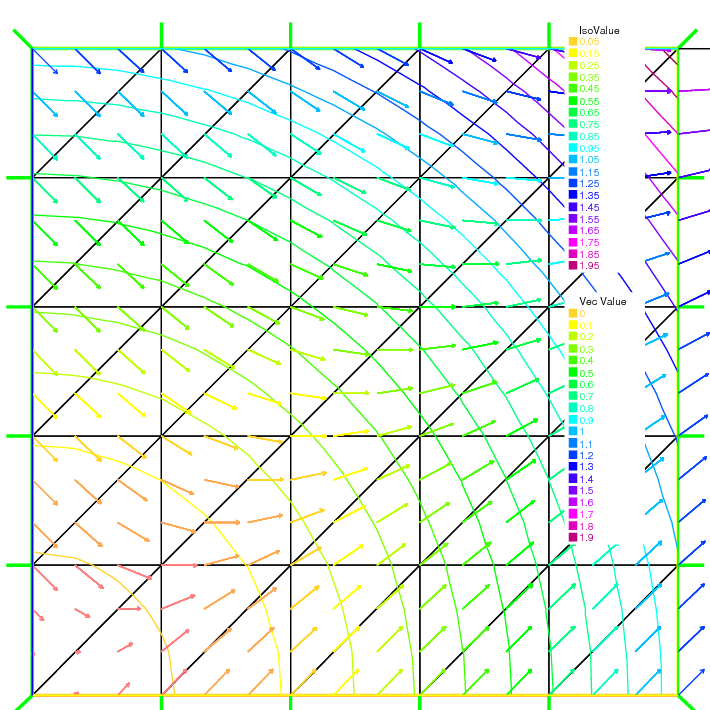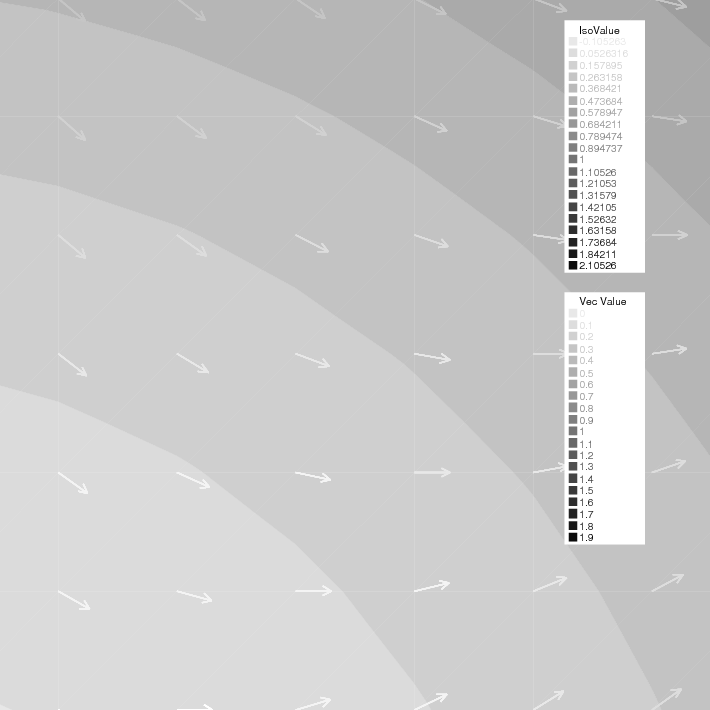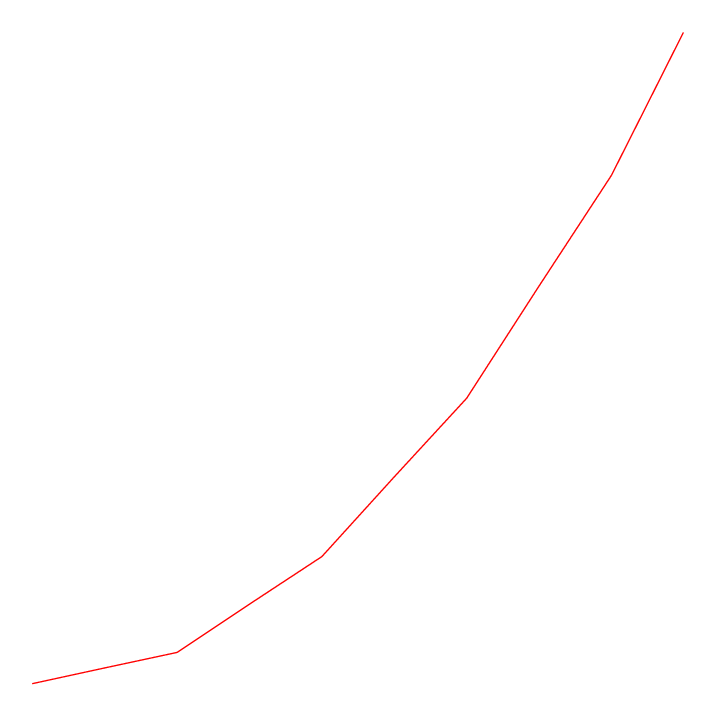FreeFEM Documentation on GitHub

stars - forks

# Visualization

Results created by the finite element method can be a huge set of data, so it is very important to render them easy to grasp.

There are two ways of visualization in FreeFEM:

• One, the default view, which supports the drawing of meshes, isovalues of real FE-functions, and of vector fields, all by the command plot (see Plot section below). For publishing purpose, FreeFEM can store these plots as postscript files.

• Another method is to use external tools, for example, gnuplot (see Gnuplot section, medit section, Paraview section, Matlab/Octave section) using the command system to launch them and/or to save the data in text files.

## Plot

With the command plot, meshes, isovalues of scalar functions, and vector fields can be displayed.

The parameters of the plot command can be meshes, real FE functions, arrays of 2 real FE functions, arrays of two double arrays, to plot respectively a mesh, a function, a vector field, or a curve defined by the two double arrays.

Note

The length of an arrow is always bound to be in [5‰, 5%] of the screen size in order to see something.

The plot command parameters are listed in the Reference part.

The keyboard shortcuts are:

• enter tries to show plot

• p previous plot (10 plots saved)

• ? shows this help

• +,- zooms in/out around the cursor 3/2 times

• = resets the view

• r refreshes plot

• up, down, left, right special keys to tanslate

• 3 switches 3d/2d plot keys :

• z,Z focal zoom and zoom out

• H,h increases or decreases the Z scale of the plot

• mouse motion:

• left button rotates

• right button zooms (ctrl+button on mac)

• right button +alt tanslates (alt+ctrl+button on mac)

• a,A increases or decreases the arrow size

• B switches between showing the border meshes or not

• i,I updates or not: the min/max bound of the functions to the window

• n,N decreases or increases the number of iso value arrays

• b switches between black and white or color plotting

• g switches between grey or color plotting

• f switches between filling iso or iso line

• l switches between lighting or not

• v switches between show or not showing the numerical value of colors

• m switches between show or not showing the meshes

• w window dump in file ffglutXXXX.ppm

• * keep/drop viewpoint for next plot

• k complex data / change view type

• ESC closes the graphics process before version 3.22, after no way to close

• otherwise does nothing

For example:

 1real[int] xx(10), yy(10);
2
3mesh Th = square(5,5);
4
5fespace Vh(Th, P1);
6
7//plot scalar and vectorial FE function
8Vh uh=x*x+y*y, vh=-y^2+x^2;
9plot(Th, uh, [uh, vh], value=true, ps="three.eps", wait=true);
10
11//zoom on box defined by the two corner points [0.1,0.2] and [0.5,0.6]
12plot(uh, [uh, vh], bb=[[0.1, 0.2], [0.5, 0.6]],
13   wait=true, grey=true, fill=true, value=true, ps="threeg.eps");
14
15//compute a cut
16for (int i = 0; i < 10; i++){
17   x = i/10.;
18   y = i/10.;
19   xx[i] = i;
20   yy[i] = uh; //value of uh at point (i/10., i/10.)
21}
22plot([xx, yy], ps="likegnu.eps", wait=true);Fig. 112 Mesh, isovalue and vectorFig. 113 Enlargement in grey of isovalue and vectorFig. 114 Plots a cut of uh. Note that a refinement of the same can be obtained in combination with gnuplot

Plot

To change the color table and to choose the value of iso line you can do:

 1// from: \url{http://en.wikipedia.org/wiki/HSV_color_space}
2// The HSV (Hue, Saturation, Value) model defines a color space
3// in terms of three constituent components:
4// HSV color space as a color wheel
5// Hue, the color type (such as red, blue, or yellow):
6// Ranges from 0-360 (but normalized to 0-100% in some applications, like here)
7// Saturation, the "vibrancy" of the color: Ranges from 0-100%
8// The lower the saturation of a color, the more "grayness" is present
9// and the more faded the color will appear.
10// Value, the brightness of the color: Ranges from 0-100%
11
12mesh Th = square(10, 10, [2*x-1, 2*y-1]);
13
14fespace Vh(Th, P1);
15Vh uh=2-x*x-y*y;
16
17real[int] colorhsv=[ // color hsv model
18   4./6., 1 , 0.5, // dark blue
19   4./6., 1 , 1, // blue
20   5./6., 1 , 1, // magenta
21   1, 1. , 1, // red
22   1, 0.5 , 1 // light red
23   ];
24 real[int] viso(31);
25
26 for (int i = 0; i < viso.n; i++)
27   viso[i] = i*0.1;
28
29 plot(uh, viso=viso(0:viso.n-1), value=true, fill=true, wait=true, hsv=colorhsv);